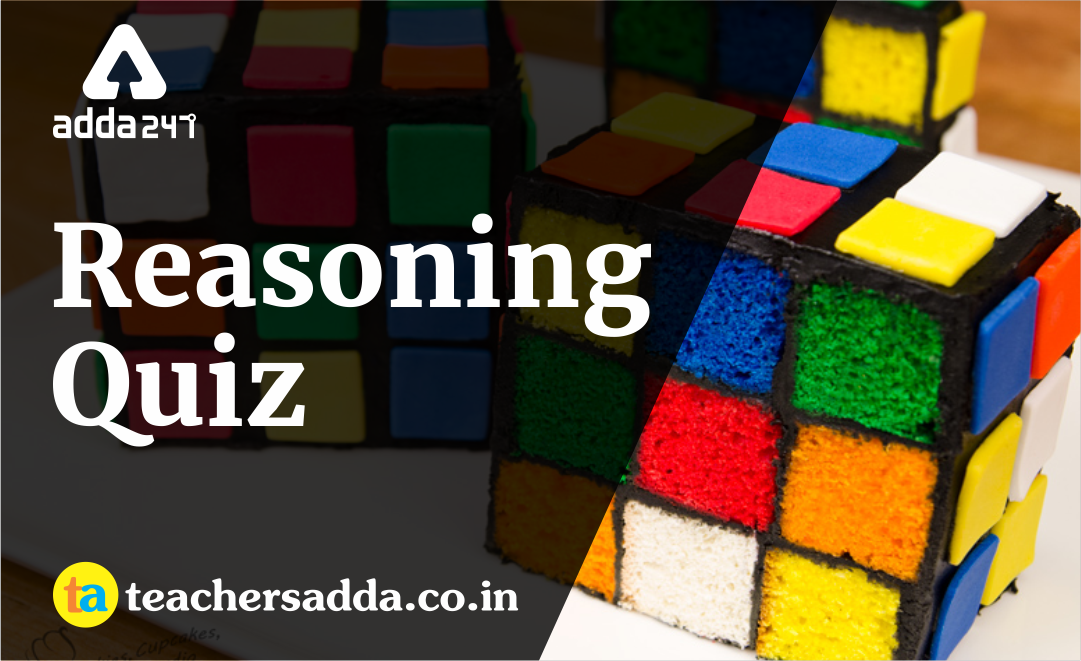The reasoning is the most scoring section in any competitive exam. It also develops or nourishes our critical thinking skills as compared to other subjects. In DSSSBKVSAPS Exam as a student to score well in this part, you have to start your preparation now because there is negative marking as well. The more you have prepared, the better you score.

Q1. If A x B means A is the brother of B and A + B means that A is the father of B then which one means that ‘M is the nephew of N’?
(a) N + MxK
(b) NxK+M
(c) MxK+N
(d) None of the above

Q2. Which diagram best describes the relationship between Human beings, Daughters, Mothers?Q3. Which word cannot be formed from the letters of the word ADMINISTRATION, using each letter only once?
(a) SITUATION
(b) STRAIN
(d) RATION

Q4. If ‘+’ means ‘÷’, ‘x’ means ‘-’ ‘÷’ means ‘+’ and ‘-’ means ‘x’, then
16 ÷ 8 x 6 – 2 + 12 =?
(a) 23
(b) 24
(c) 17
(d) 22

Q5. If 17 x 64 = 4716, 28 x 34 = 4823 then 76 x 84 =?
(a) 6487
(b) 8764
(c) 4678
(d) 4768

Q6. Find the missing term. 25, 36, 49, 64, 81,?
(a) 97
(b) 100
(c) 105
(d) 121

Q7. If BARLEY is written as CBTMGZ, SOUND will be written as
(a) URWOF
(b) TPWOF
(c) UPWPF
(d) None of the above

Q8. Complete the series. BMK DLM FKO HJQ?
(a) JHS
(b) JIS
(c) JGK
(d) JIR

Direction (Q9-10):  In each question, Figure 2 has a certain relation to Figure 1. Select the option for Figure 4 which has the same relation to Figure 3 as Figure 2 has to Figure 1.

Q9.Q10.Solutions

S1. Ans.(b)
Sol.
K + M → K is father of M.
N × K → N is brother of K
∴ M is cousin of N.

S2. Ans.(d)
Sol.S3. Ans.(a)
Sol.
‘u’ is extra in situation.

S4. Ans.(a)
Sol.
16 ÷ 8 × 6 – 2 = 16 + 8 – 6 × 2/12
= 16 + 8 – 1 = 23

S5. Ans.(c)
Sol.
17 × 64 → 4716
12    34 → 4213
28 × 34 → 4823
12    34      4213
76 × 84 → 4678
12    34 → 4213

S6. Ans.(b)
Sol.S7. Ans.(b)
Sol.S8. Ans.(b)
Sol.S9. Ans.(d)
Sol.

S10. Ans.(b)
Sol.

You may also like to read :